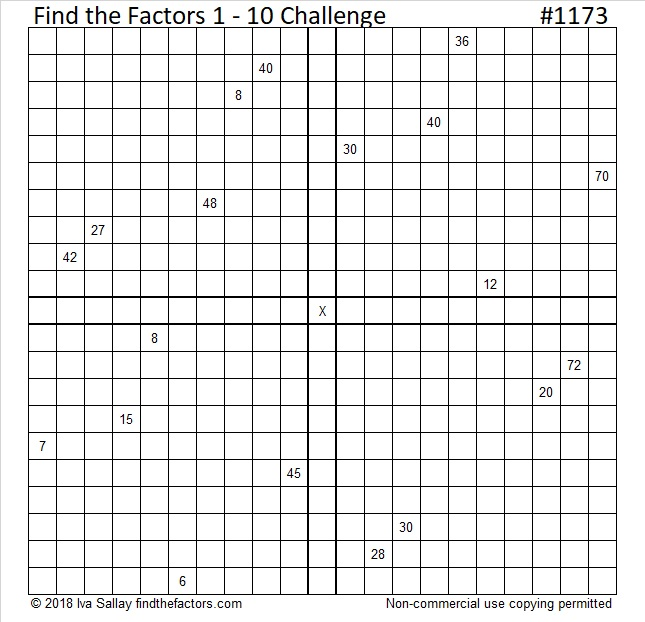# 1173 Challenge Puzzle

Getting started on this Challenge Puzzle will take some thinking, but solving it is worth all the effort. Remember use logic, not guess and check, and you will eventually be successful!Print the puzzles or type the solution in this excel file: 12 factors 1161-1173

Here’s some information about the number 1173:

• 1173 is a composite number.
• Prime factorization: 1173 = 3 × 17 × 23
• The exponents in the prime factorization are 1, 1, and 1. Adding one to each and multiplying we get (1 + 1)(1 + 1)(1 + 1) = 2 × 2 × 2 = 8. Therefore 1173 has exactly 8 factors.
• Factors of 1173: 1, 3, 17, 23, 51, 69, 391, 1173
• Factor pairs: 1173 = 1 × 1173, 3 × 391, 17 × 69, or 23 × 51
• 1173 has no square factors that allow its square root to be simplified. √1173 ≈ 34.249091173 is the hypotenuse of a Pythagorean triple:
552-1035-1173 which is (8-15-17) times 69

1173 is palindrome 3B3 in BASE 18 (B is 11 base 10)
because 3(18²) + 11(18) + 3(1) = 1173

This site uses Akismet to reduce spam. Learn how your comment data is processed.